# 5 Ways to Find Matching Values in Two Worksheets in Excel

Very often we need to deal with multiple worksheets with a good deal of similar values. From the lengthy spreadsheets, it becomes difficult for us to extract duplicate values. So, to eliminate this difficulty, this blog will guide you with 5 quick and easy ways to find matching values in two worksheets in Microsoft Excel.

## Introduction to the Dataset

I will be using two sheets (List 1 and List 2) with slight differences in their cell values. There is a list of passenger names, their boarding counters, flight numbers, and their cellphone numbers.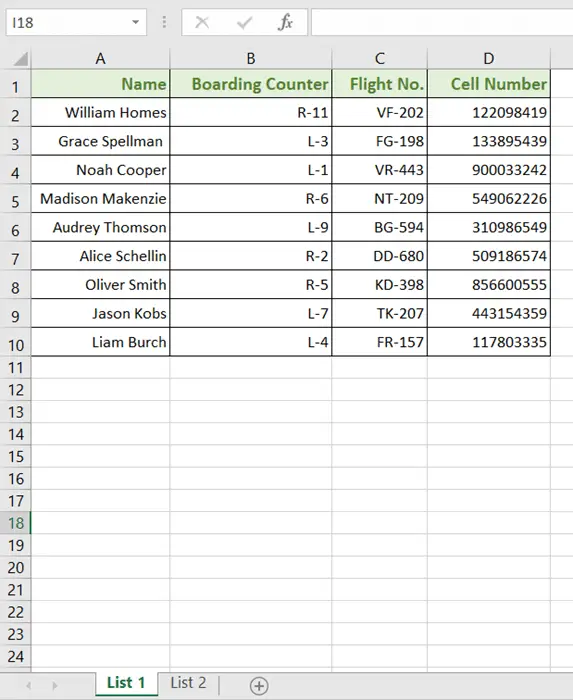## Easiest Way to Find Matching Values in Two Worksheets

You can find the matching values of two separate worksheets by using the EXACT function in Microsoft Excel. It will return either TRUE or FALSE. I like to remind you that the EXACT function works case-sensitively.

Here is the syntax of the formula 🡻

`EXACT(Text1, Text2)`

Step_1: First, create a separate column beside the data table in one of the sheets.

I’ve inserted a column in Column E, named ‘Results’.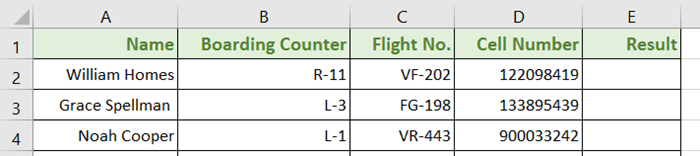Step_2: Then insert the formula in the first empty cell of the new column (E2):

`=EXACT(D2:D10,'List 2'!D2:D10)`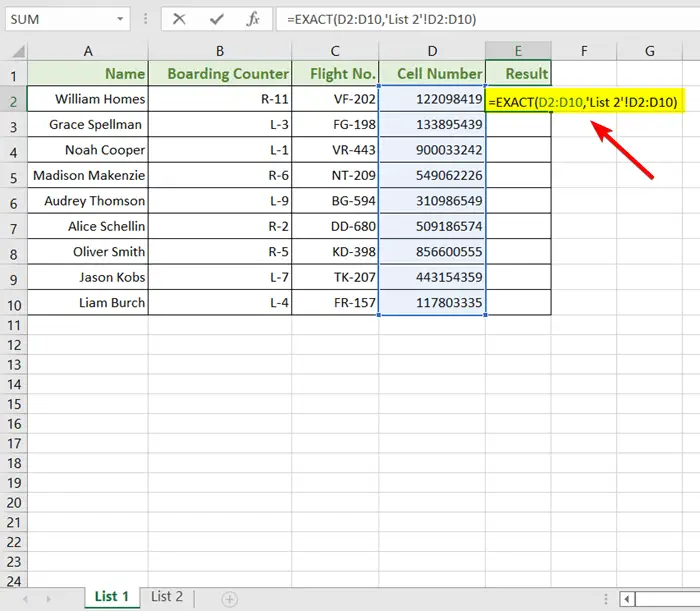In the formula above,
• The first range, D2:D10 is from the List 1 sheet.
• ‘List 2’! Is the second sheet.
• D2:D10 is the range from the sheet, “List 2”.

Step_3: Then, press ENTER to apply the formula.

Step_4: Now double-click on the Fill Handle, the ‘+’ sign on the bottom-right corner of cell E2.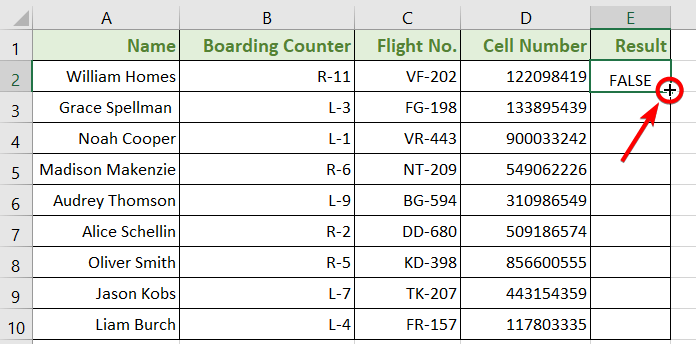Final Result 🡻

The Fill Handle will automatically copy the formula through the whole datasheet and show the results this way: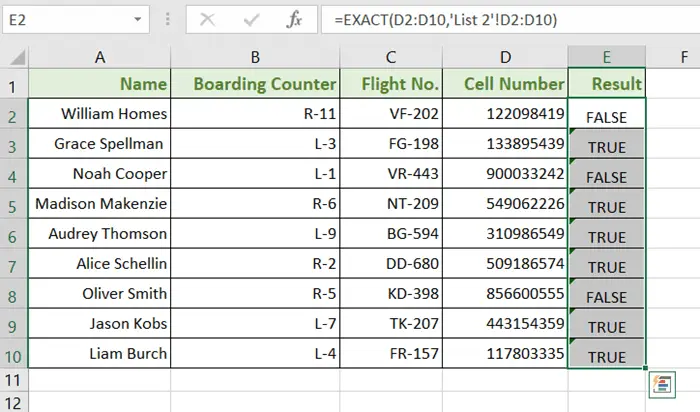🔗 Vlookup for Duplicate Values and Return the Matches in Excel [8 Cases]

🔗 How to Find Duplicates in Two Columns in Excel (7 Methods)

### Alternative Way #1: Use Conditional Formatting to Find Matching Values in Two Excel Worksheets

Conditional Formatting is a great tool to mark data with different types of highlights. I will be using it to highlight the matches. But before using the Conditional Formatting command, I will apply the COUNTIF function to detect the identical values from two sheets,

Here is the syntax of the formula 🡻

`COUNTIF(CELL RANGE, CRITERIA)`

Cell Range is the set of cells.

Criteria are our targeted value.

Step_1: Select your target cell range.

I have selected the column, Boarding Counter (Column B) and Flight No. (Column C) from the List 1 sheet.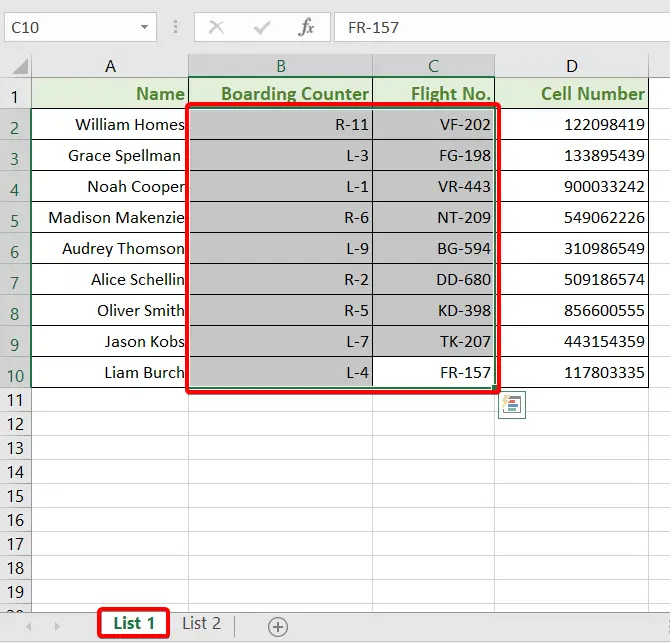Step_2: Then go to the Home tab.

Step_3: Now hit on the Conditional Formatting drop-down list from the Styles group.

Step_4: Select the New Rules command from the menu.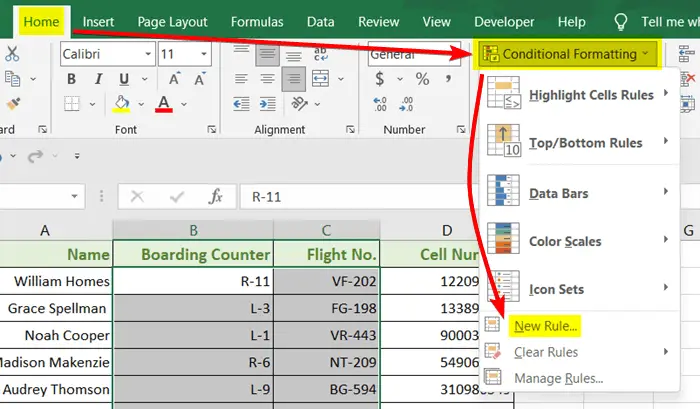The New Formatting Rules dialog box will pop up on the sheet.

Step_5: From the Rule Type section, choose the Use a Formula to Determine Which Cells to Format option.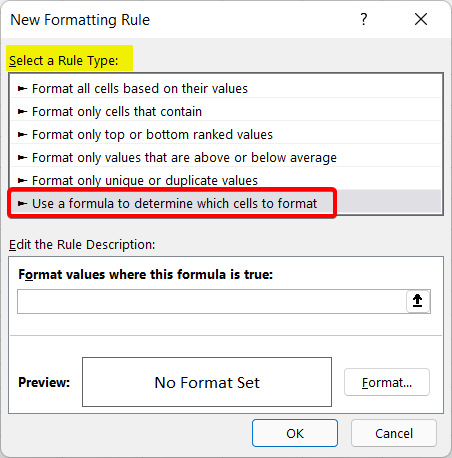Step_6: Then write down the following formula in the Format Values Where This Formula is True bar:

`=COUNTIF('List 2'!\$B\$2:\$C\$10,B2)`
In the formula above,
• ‘List 2’! is the second sheet.
• \$B\$2:\$C\$10 is the absolute cell reference of the cell range B2:C10 of List 2.
• Absolute cell reference keeps the cells locked. Add the dollar (\$) sign before each character to apply absolute cell reference.
• B2 is the first cell that is working as the criteria. Make sure you do not put a dollar (\$) sign when writing the criteria.

Step_7: Hit the FORMAT button on the right-bottom side of the box.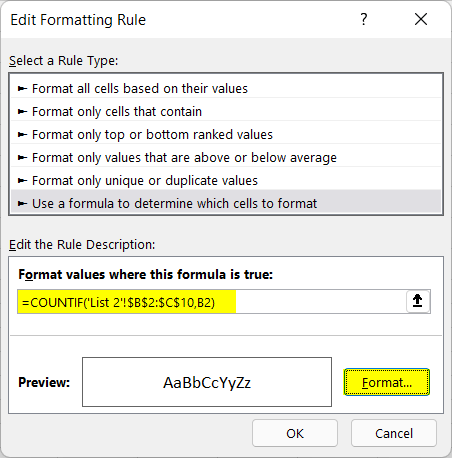The Format Cells dialog box will show up.

Step_8: Now from the Fill section, choose a Background Color.

I have chosen the light orange color.

Step_9: Hit OK to see the changes.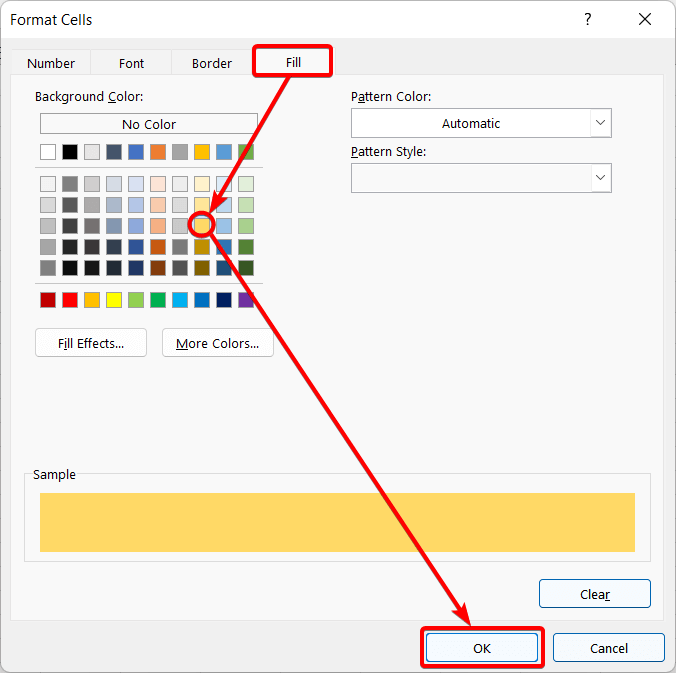Final Result 🡻

The similar cells between List 1 and List 2 are now highlighted with orange color now.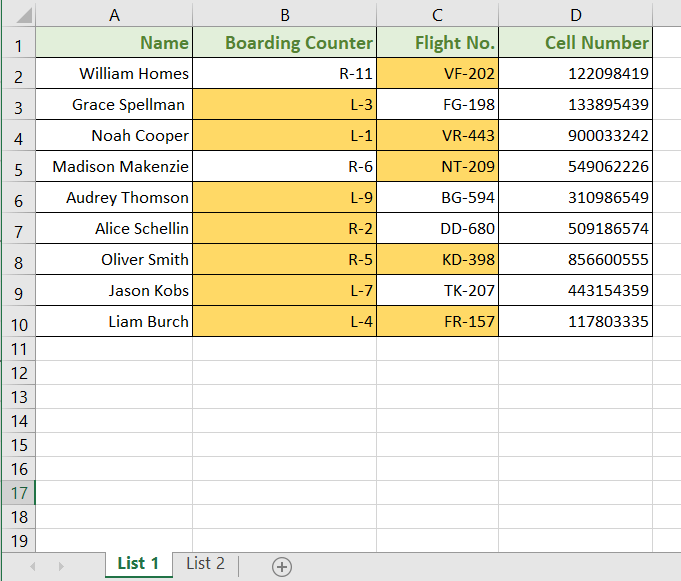🔗 How to Compare Rows in Excel for Duplicates (7 Ways)

### Alternative Way #2: Combine MATCH and ISNUMBER Function to Find Matching Values in Two Worksheets in Excel

This method is another way to find the matching values in a TRUE-FALSE manner. But this time we will use the MATCH function and ISNUMBER function.

Here is the syntax of the formula 🡻

`ISNUMBER(MATCH(lookup_value, lookup_array, [match_type])`

The MATCH function detects the location of the identical values. And the ISNUMBER function gives an output of TRUE (for matches) and FALSE (for mismatches) according to the results.

I am going to find similar texts in Column C from both sheets.

Step_1: Insert a new column beside the data table in one of the sheets.

I’ve inserted a column in Column E, named ‘Findings’.

Step_2: Then inside the E2 cell, write down this formula:

`=ISNUMBER(MATCH(C2,'List 2'!C2:C10,0))`
In the formula above,
• C2 is the lookup_value. It is the value of the first cell of my targeted column.
• ‘List 2’! C2:C10 is the lookup_array that selects the C2 to C10 range from the List 2 sheet.
• 0 defines the difference between lookup_value and lookup_array.

Step_3: Press ENTER to see the results.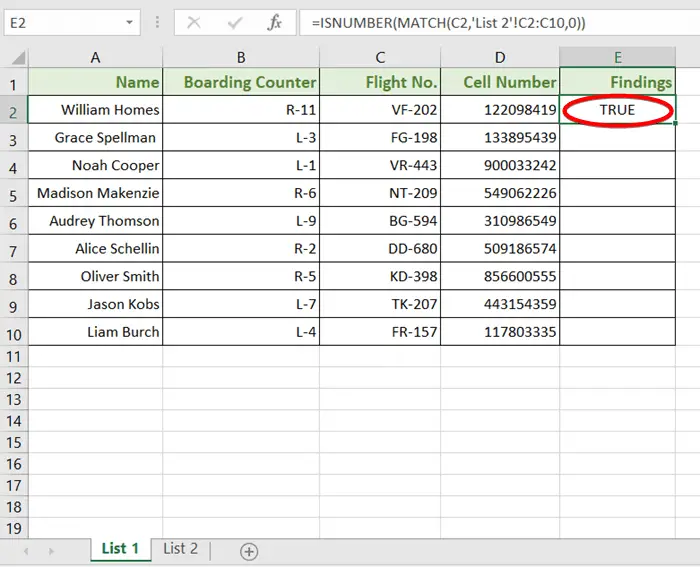Step_4: Now bring the cursor to the bottom-right corner of cell E2 and double click on the Fill Handle icon.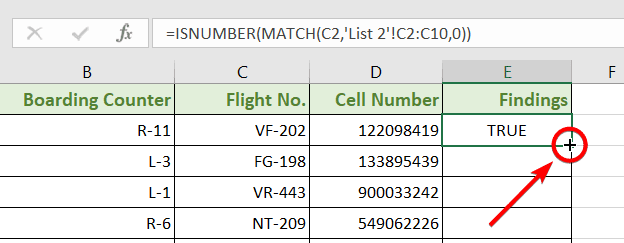Final Result 🡻

The TRUE-FALSE result approaches the present and absence of similarity in these two sheets. TRUE means, the values of two sheets match, and FALSE means the values are unique.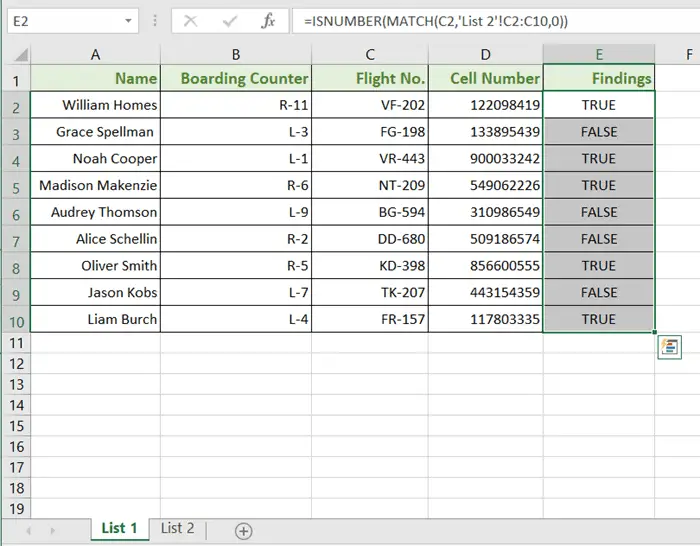🔗 How to Find Similar Text in Two Columns in Excel (5 Ways)

🔗 5+ Formulas to Find Duplicates in One Column in Excel

### Alternative Way #3: Apply VLOOKUP Function to Find Matching Values in Two Worksheets in Excel

The VLOOKUP function can find matching data from two sheets and extract them out of any row or column. In this method, I will bring out similar values in a new column.

You can also check out this: VLOOKUP for Duplicate Values and Return the Matches [8 Cases].

Here is the syntax of the formula 🡻

`VLOOKUP(lookup_value, table_array, col_index_number, [range_lookup])`

Step_1: Create a new column beside the existing columns.

I inserted a column named ‘Matching Values’ in Column E.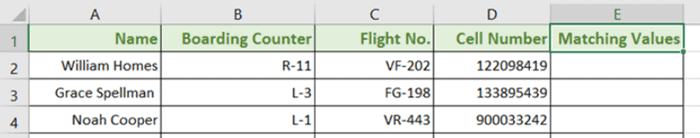Step_2: Then copy the following formula in the top cell (E2) of that column:

`=VLOOKUP(D2,'List 2'!\$D\$2:\$D\$10,1,FALSE)`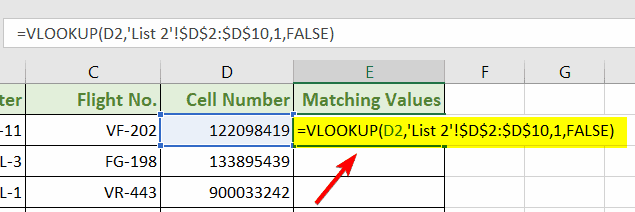In the formula above,
• D2 is the lookup value which is the top cell of the column Cell Number.
• \$D\$2:\$D\$10 is the table array which is the range of the column Cell Number from the List 2 worksheet.
• 1 is the column index number.
• FALSE finds the exact matches from the two worksheets.

Step_3: Press ENTER to apply the formula.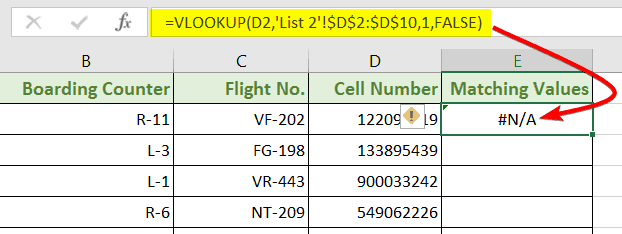Step_4: Now bring the cursor to the bottom-right corner of cell E2 and double click on the Fill Handle icon.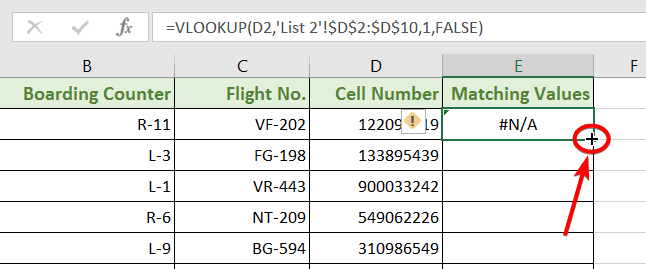Final Result 🡻

The Matching Values column now exhibits similar values from Column D of both sheets. Some of the results came #N/A, which means the values of those cells didn’t match with the other sheet.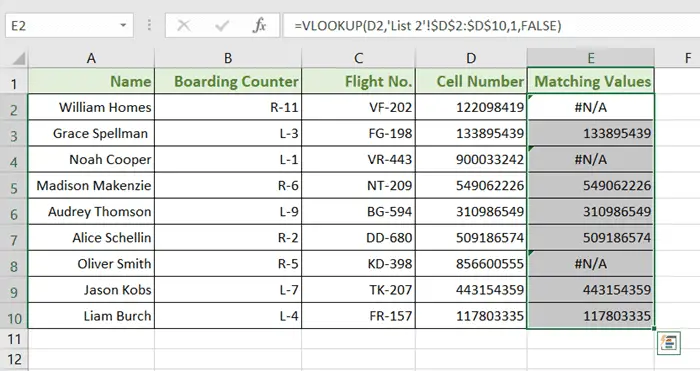🔗Find, Highlight, and Remove Duplicates in Excel [Step-by-Step]

🔗 4+ Methods to Filter Duplicate Values in Excel

### Alternative Way #4: Use IF and ISNA Functions to Find Matching Values in Two Worksheets in Excel

I will be using the IF function, which compares the selected cell range. Another function, the ISNA function shows ‘TRUE’ if it finds #N/A. Otherwise, it leaves ‘FALSE’ if it finds other values.

Step_1:  Create a new column beside the existing columns.

I inserted a column named ‘Duplicate Values’ in Column E.

Step_2: Then copy the following formula in the top cell (E2) of that column:

`=IF(ISNA(VLOOKUP(B2,'List 2'!\$B\$2:\$D\$10,1,FALSE)),"NO","YES")`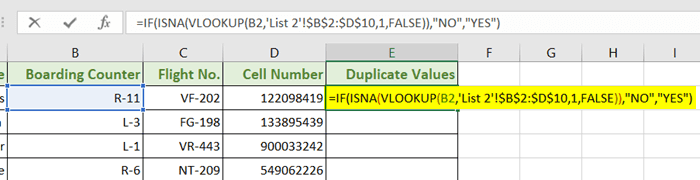In the formula above,
• B2 is the lookup value.
• \$B\$2:\$D\$10 is the table array which is the range of the 3 columns (Boarding Counter, Flight No, and Cell Number) from the List 2 worksheet.
• 1 is the column index number.
• FALSE finds similar characters from the two worksheets.
• “NO” and “YES” are the outputs.

Step_3: Press ENTER to apply the formula.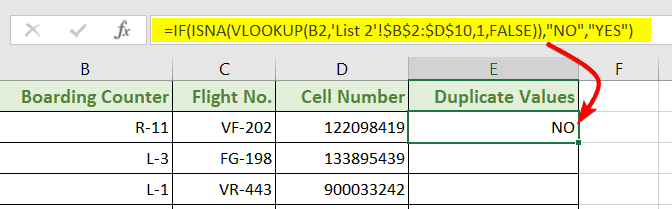Step_4: Now bring the cursor to the bottom-right corner of cell E2 and double click on the Fill Handle icon.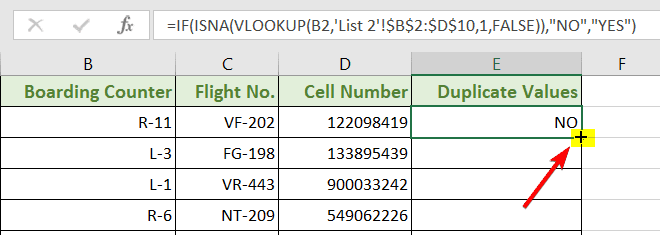Final Result 🡻

The duplicate values resulted in YES, and the different values resulted in NO.

##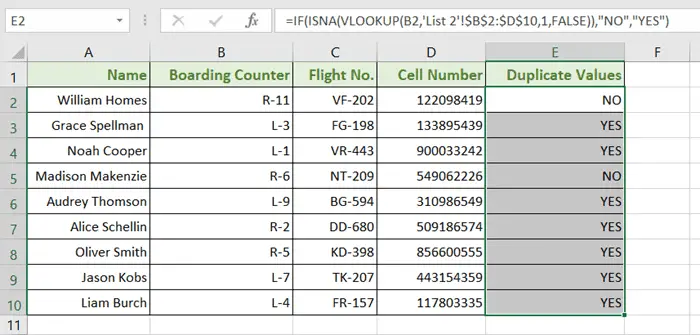🔗 How to Remove Duplicates in Excel [13 + Different Methods]

🔗 4+ Ways to Find Duplicates in a Column and Delete Rows in Excel

## Conclusion

I hope you will face no trouble finding similar data in Excel worksheets from now. Feel free to comment below if you have any confusion or any suggestion for us. Also, let us know if you want to share any easier techniques with us.

(Visited 1,526 times, 8 visits today)